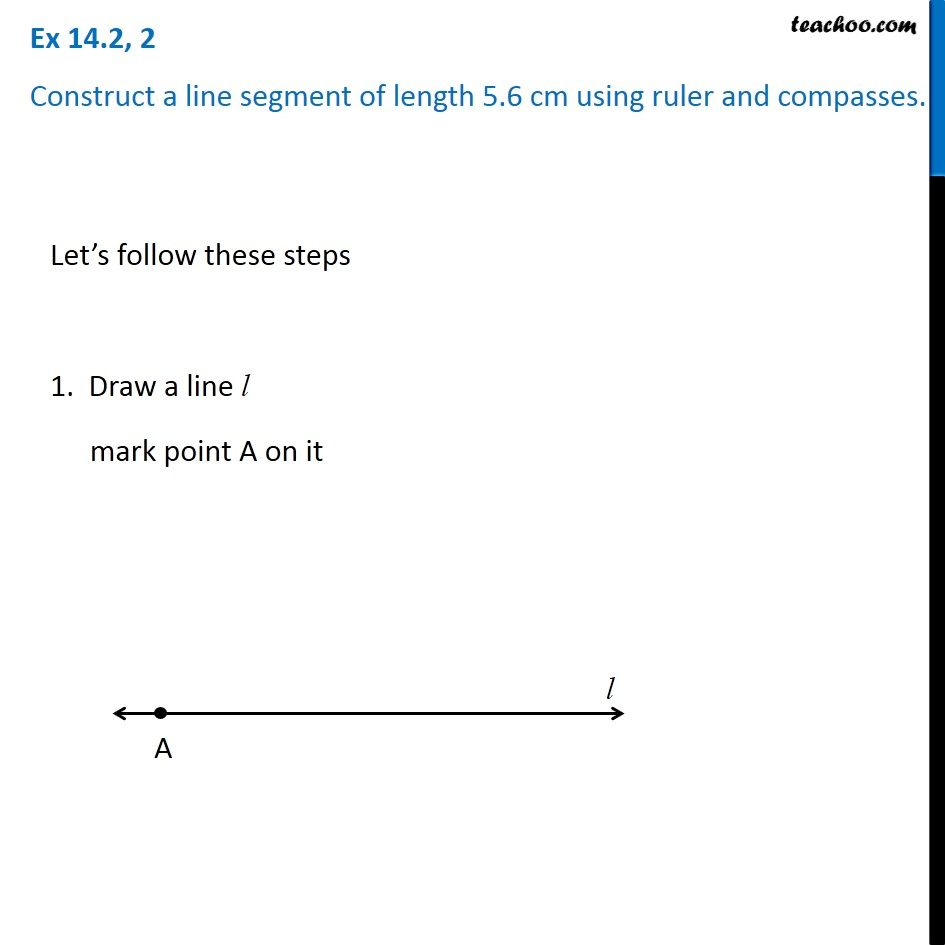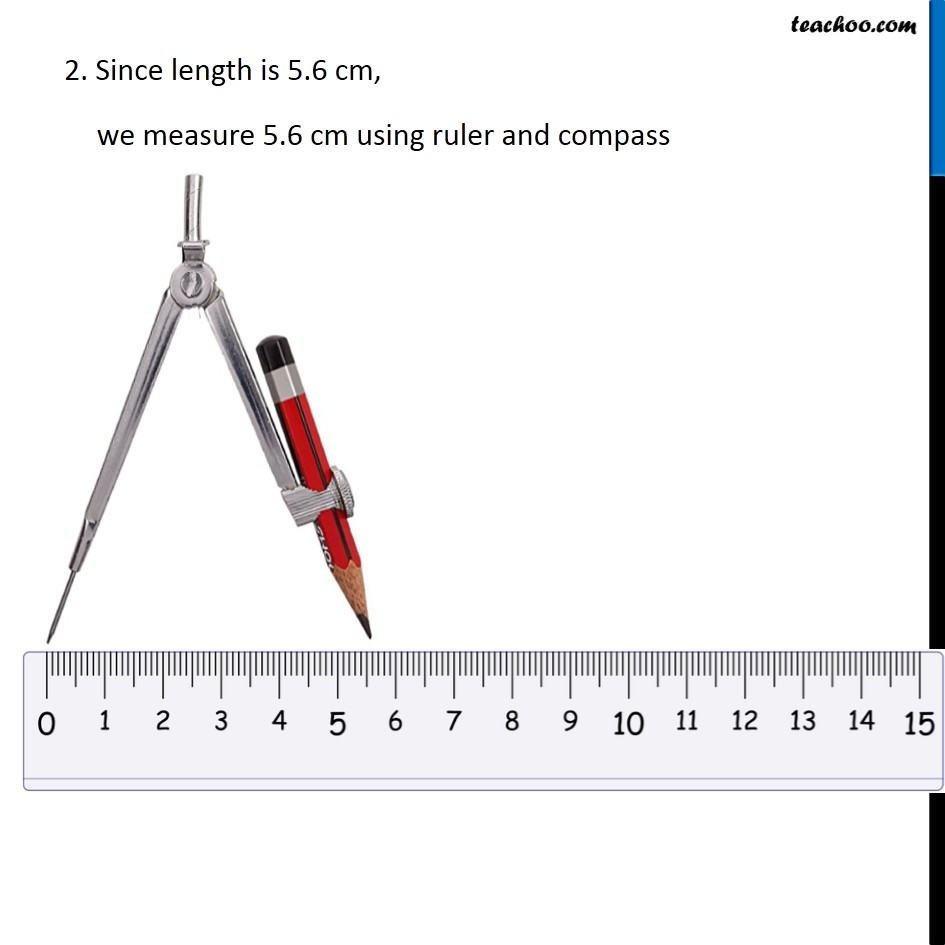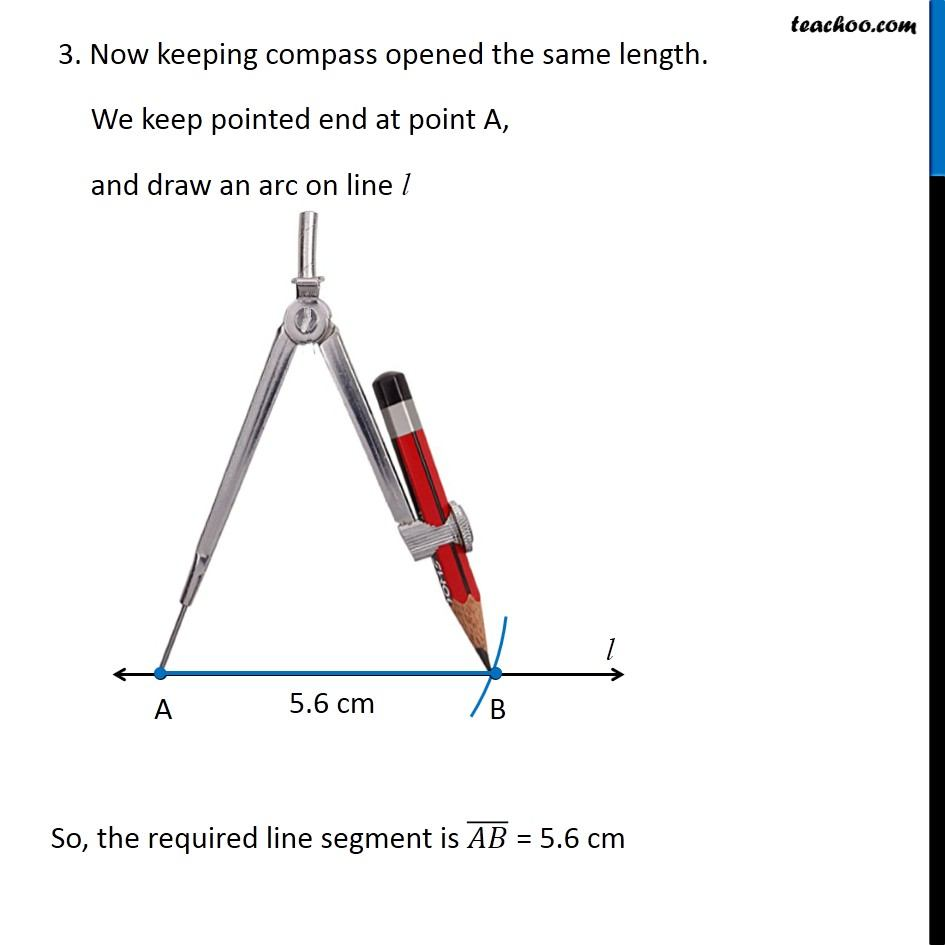Subscribe to our Youtube Channel - https://you.tube/teachoo

1. Chapter 14 Class 6 Practical Geometry
2. Concept wise
3. Construction of a line segment of a given length

Transcript

Ex 14.2, 2 Construct a line segment of length 5.6 cm using ruler and compasses. Let’s follow these steps 1. Draw a line l mark point A on it 2. Since length is 5.6 cm, we measure 5.6 cm using ruler and compass 2. Since length is 5.6 cm, we measure 5.6 cm using ruler and compass 2. Since length is 5.6 cm, we measure 5.6 cm using ruler and compass So, the required line segment is (𝐴𝐵) ̅ = 5.6 cm

Construction of a line segment of a given length